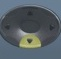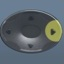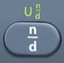## Cone

Cone = Three-Dimensional Shape with Circular Base Connecting to a PointBasics
r = radius = distance halfway across circular base of cone
d = diameter = distance all the way across circular base of cone
h = height
s = slant height = distance along cone’s side
Apex = point of cone
Base = circular portion of cone
SA = Surface Area = area covering surface, including base, of cone (measured in square units)
LA = Lateral Area = area covering surface, excluding base, of cone (measured in square units)
V = Volume = space occupied by cone (measured in cubic units)

Formulas (provided by Mathematics Formula Sheet)

SA = πrs + πr²

V =$\bf\displaystyle\frac{1}{3}$πr²h

Formulas (NOT provided by Mathematics Formula Sheet)

LA = πrs$\bf\displaystyle r=\frac{1}{2}d$

Question
A solid highway cone has a radius of 6 inches, height 8 inches, and slant height 10 inches.
What is its surface area?

96π square inches

SA = πrs + πr²
SA = π × 6 × 10 + π × 6² = 96π

Calculator Click What You See Commentblinker clears screen
π × 6 × 10 + π × 6π*6*10+π*6² SA = πrs + πr²96π Answer
###### Cone

Question

With reference to the question above, what is the area of the cone in decimal form?

301.5928947 square inches

See Toggle.
96π ⇔ 301.5928947

Calculator Click What You See Commentblinker clears screen
π × 6 × 10 + π × 6π*6*10+π*6² SA = πrs + πr²96π301.5928947 Answer
###### Cone

Question

With reference to the question above, what is the area of the cone to the nearest tenth?

301.6 square inches

See Rounding.
301.5928947  301.6

Question
A solid highway cone has a radius of 6 inches, height 8 inches, and slant height 10 inches.
To the nearest tenth of a square foot, what is its surface area?

2.1 square feet

See Measurement.
Square Feet = Square Inches ÷ 144
Square Feet = 301.5928947 ÷ 144 = 2.094395102 = 2.1 after rounding

Calculator Click What You See Commentblinker clears screen
π × 6 × 10 + π × 6π*6*10+π*6² SA = πrs + πr²96π301.5928947
301.5928947 ÷ 144 301.5928947÷144 Square Feet =
Square Inches ÷ 1442.094395102 Answer
(before rounding)
###### Cone

Question
A solid toy cone has a radius of 3 inches, height 4 inches, and slant height 4 inches.
To the nearest hundredth of a cubic inch, what is its volume?

37.70 cubic inches

V =$\bf\displaystyle\frac{1}{3}$πr²h

V =$\bf\displaystyle\frac{1}{3}$*π*3²*4 = 37.69911184 after toggle = 37.70 after rounding

Calculator Click What You See Commentblinker clears screen13×
π × 3× 4$\bf\displaystyle\frac{1}{3}$*π*3²*4 V =$\bf\displaystyle\frac{1}{3}$πr²h12π37.69911184 Answer
(before rounding)
###### Cone

Question
A solid highway cone has a diameter of$\bf\displaystyle6\frac{1}{2}$ inches, height$\bf\displaystyle4\frac{1}{4}$ inches, and slant height$\bf\displaystyle5\frac{3}{4}$inches.  To the nearest hundredth of a cubic inch, what is its volume?

47.01 cubic inches$\bf\displaystyle r=\frac{1}{2}d$$\bf\displaystyle r=\frac{1}{2}$*$\bf\displaystyle 6\frac{1}{2}$

V =$\bf\displaystyle\frac{1}{3}$πr²h

V =$\bf\displaystyle\frac{1}{3}$*π*$\bf\displaystyle{{\frac{13}{4}}^{2}}$*$\bf\displaystyle 4\frac{1}{4}$$\bf\displaystyle\frac{2873\pi }{192}$ = 47.00935257 after toggle = 47.01 after rounding

Calculator Click What You See Commentblinker clears screen12×612$\bf\displaystyle\frac{1}{2}$*$\bf\displaystyle 6\frac{1}{2}$$\bf\displaystyle r=\frac{1}{2}d$$\bf\displaystyle\frac{13}{4}$ r13×
π ×134×414$\bf\displaystyle\frac{1}{3}$*π*$\bf\displaystyle{{\frac{13}{4}}^{2}}$*$\bf\displaystyle 4\frac{1}{4}$ V =$\bf\displaystyle\frac{1}{3}$πr²h$\bf\displaystyle\frac{2873\pi }{192}$47.00935257 Answer
(before rounding)
###### Cone

Question
A solid highway cone has a radius of 3 inches, height 4 inches, and slant height 5 inches.
To the nearest tenth of a square inch, what is its lateral area?

47.1 square inches

LA = πrs
LA = π × 3 × 5 = 47.1238898 after toggle = 47.1 after rounding

Calculator Click What You See Commentblinker clears screen
π × 3 × 5 π*3*5 LA = πrs15π47.1238898 Answer
(before rounding)
###### Cone

Practice – Questions
1.  A solid highway cone has a radius of 5 inches, height 12 inches, and slant height 13 inches.  To the nearest tenth of a square inch, what is its surface area?

2.  With reference to Question 2, what is the cone’s surface area to the nearest tenth of a square foot?

3.  A cotton candy cone has a radius of 5 inches, height 12 inches, and slant height 13 inches.  To the nearest hundredth of a cubic inch, what is its volume?

4.  A cotton candy cone has a diameter of$\bf\displaystyle10\frac{1}{2}$ inches, height$\bf\displaystyle12\frac{1}{4}$ inches, and slant height$\bf\displaystyle13\frac{3}{4}$ inches.  To the nearest hundredth of a cubic inch, what is its volume?

5.  A cotton candy cone has a radius of 5 inches, height 12 inches, and slant height 13 inches.  To the nearest tenth of a square inch, what is its lateral area?